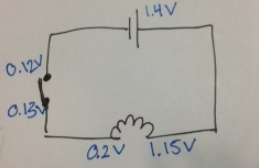# Problem: A. How much voltage does the light bulb uses up? How do you measure this value?B. What is the change in the potential energy of a single electron as it moves through the light bulb?C. Is this a gain or loss of potential energy?D. Where in the light bulb do you think this gain or loss occurs? Explain your reasoning.

###### FREE Expert Solution

We are required to explain the dissipation of electrical energy in a circuit.

90% (297 ratings)###### Problem Details

A. How much voltage does the light bulb uses up? How do you measure this value?

B. What is the change in the potential energy of a single electron as it moves through the light bulb?

C. Is this a gain or loss of potential energy?

D. Where in the light bulb do you think this gain or loss occurs? Explain your reasoning.